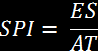### Schedule Performance Index & Schedule Variance

A project's Schedule Variance (SV) is simply the difference between the Earned Value (EV) and the Planned Value (PV). The value is positive if the budgeted cost of the work performed is greater than the budgeted cost of the work scheduled. In other words, SV is positive whether a project is ahead of schedule.Click to maximize

The Schedule Performance Index (SPI) commonly is defined as the ratio of Earned Value (EV) to the Planned Value (PV). The value is greater than one if the budgeted cost of the work performed is greater than the budgeted cost of the work scheduled. In other words, SPI is greater than one whether a project is ahead of schedule. As I explained in a last post (http://www.earned-value.com/2011/03/spi-is-not-good-index-at-project-finish.html), the SPI, defined in this way, is less effective when a project nears the end. It is clear that when EV is close to PV, the indicator tends to 1, which is the ideal value. Even when a project is completed late, the SPI indicator always ends in 1. A better way to define the SPI is using the concept of Earned Schedule (ES) as I defined in an last post (http://www.earned-value.com/2011/03/practical-estimation-of-earned-schedule.html). Then, considering AT being the Actual Time, SPI could be better defined as the folowing formula.Click to maximize
The SPI is useful as a measure of the past performance of the project in the time dimension. It can be used to forecast the duration (D) at completion of a project using the scheduled total duration (T), as the folowing formula shows.Click to maximize